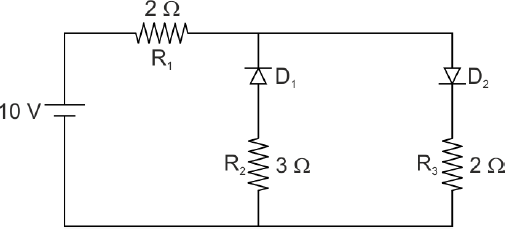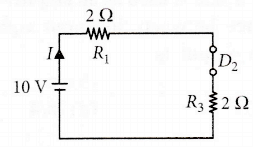#### The given circuit has two ideal diodes connected as shown in the figure below. The current flowing through the resistance R1 will beOption 1) 2.5 A Option 2) 10.0 A Option 3) 1.43 A Option 4) 3.13 AP -N junction as diode -

It is a one way device. It offers a low resistance when forward biased and high resistance when reverse biased.

- wherein

R = 0, ForwardReverse

In the given figureis in Reverse biased mode. hence no current win flow through it whileis in forward biased mode hence will offer Zero resistanceI =Correct Option isOption 1)

2.5 A

This option is incorrect.

Option 2)

10.0 A

This option is incorrect.

Option 3)

1.43 A

This option is incorrect.

Option 4)

3.13 A

This option is incorrect.

## Crack NEET with "AI Coach"

• HD Video Lectures
• Unlimited Mock Tests
• Faculty SupportDiode D1 is reverse biased so, it will block the current and diode D2 is forward biased, so it will pass the current.  Hence, the equivalent circuit becomes as shown in the figure.Current in the circuit: Current flowing through the resistance

V=IR

I= V/R = 10/2+2 = 2.5 AMPERE